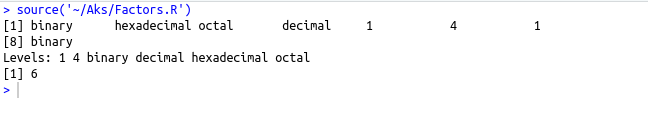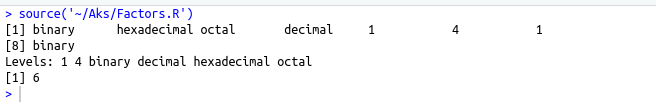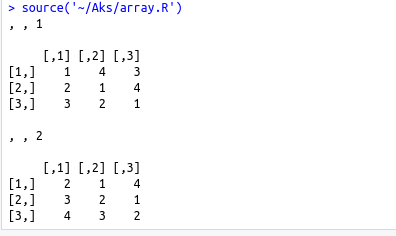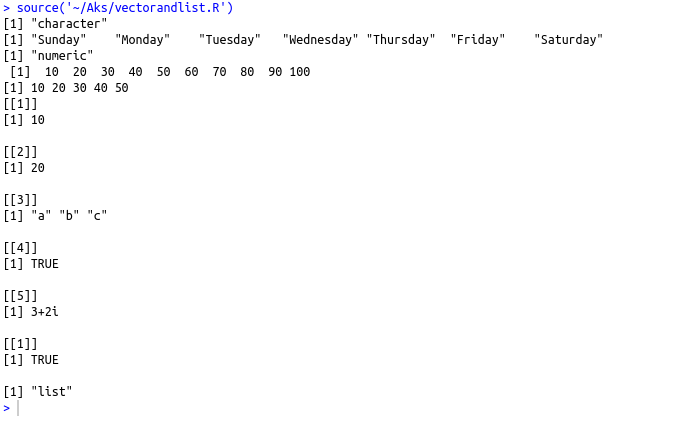• #5, First Floor, 4th Street Dr. Subbarayan Nagar Kodambakkam, Chennai-600 024 Landmark : Samiyar Madam
• pro@slogix.in
• +91- 81240 01111

### What are the Data types in R?

###### Description

Different data types and their creation

###### Process
• Vectors
• Lists
• Matrices
• Arrays
• Factors
• Data frames

Vector :

• To create vector with more than one element use the c() function
• Days<-(‘Sunday’,’Monday’,…………,’Saturday’)
Multi_of_tens=c(10,20,30,……,100)

To slice vector :

• Multi_of_tens[1:5]

List :

• Contains different types of elements in it like vector,functions etc
• List1<-list(10,20,c(‘a’,’b’,’c’),TRUE,3+2i)

Matrices :

• Two dimensional rectangular data set
• Created using vector input to matrix function
• M=matrix(c(1,2,3,4,5,6),nrow=2,ncol=3,byrow=TRUE)
• Byrow=TRUE means elements filled row by row
• Byrow=FALSE means elements filled column by column(default)

Arrays :

• Any no.Of dimensions
• Dim attribute-Creates the required no.of dimensions

Factors :

• Stores vectors with distinct values of the elements in the vector as labels
• Useful in statistical modelling
• Function – factor(),To count the levels – nlevel

Data frames :

• Tabular data objects
• Function – data.frame()
###### Sapmle Code

SOURCE CODE :(Vector and list)

#creating character vector

days<-c(‘Sunday’,’Monday’,’Tuesday’,’Wednesday’, ‘Thursday’,’Friday’,’Saturday’)

print(class(days))

print(days)

#creating numeric vector

multi_of_tens=c(10,20,30,40,50,60,70,80,90,100)

print(class(multi_of_tens))

print(multi_of_tens)

#Vector slicing

print(multi_of_tens[1:5])

#Creating a list

list1=list(10,20L,c(‘a’,’b’,’c’),TRUE,3+2i)

print(list1)

#indexing in list

print(list1)

print(class(list1))

Source code :(Matrices)

#creating a matrix

m=matrix(c(1,2,3,4,5,6),ncol = 2,nrow = 3,byrow=TRUE) #filling elements row by row

n=matrix(c(1,2,3,4,5,6),ncol = 2,nrow = 3,byrow=FALSE) #filling elements column by column

cat(“Elements filled in the matrix row by row\n”)

print(m)

cat(“Elements filled in the matrix column by column\n”)

print(n)

Source code :(Arrays)

# Create an array.

a print(a)

Source code :(factors)

# Create a factor object.

factor_data

# Print the factor.

print(factor_data)

print(nlevels(factor_data))

Source code :(Data frames)

#creating DATA FRAMES

emp_data=data.frame(Emp_id=c(‘ID01′,’ID02′,’ID03’),Emp_name=c(‘Aks’,’Daffy’,’Sofy’),Gender=c(‘F’,’F’,’F’), Age=c(25,2,2))

print(emp_data)

###### Screenshots